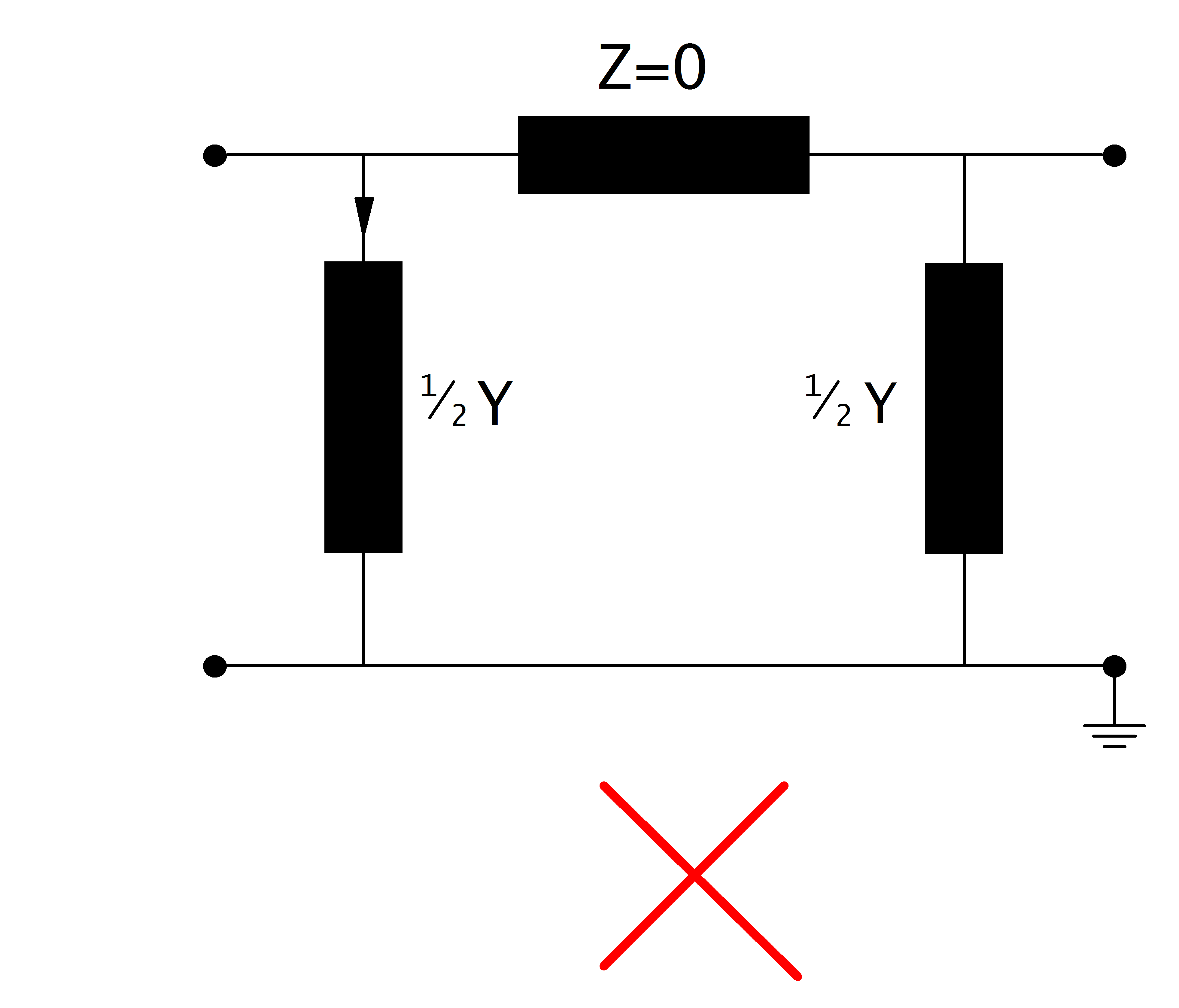Known Problems and Caveats¶

Zero Impedance Branches¶

Branches with zero impedance will lead to a non-converging power flow:This is due to the fact that the power flow is based on admittances, which would be infinite for an impedance of zero. The same problem might occur with impedances very close to zero.

Zero impedance branches occur for:

• lines with length_km = 0
• lines with r_ohm_per_km = 0 and x_ohm_per_km = 0
• transformers with vk_percent=0

If you want to directly connect to buses without voltage drop, use a bus-bus switch.